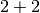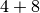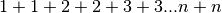This document describes Celery 2.4. For development docs, go here.

# Sets of tasks, Subtasks and Callbacks¶

New in version 2.0.

The `subtask` type is used to wrap the arguments and execution options for a single task invocation:

```subtask(task_name_or_cls, args, kwargs, options)
```

For convenience every task also has a shortcut to create subtasks:

```task.subtask(args, kwargs, options)
```

`subtask` is actually a `dict` subclass, which means it can be serialized with JSON or other encodings that doesn’t support complex Python objects.

Also it can be regarded as a type, as the following usage works:

```>>> s = subtask("tasks.add", args=(2, 2), kwargs={})

>>> subtask(dict(s))  # coerce dict into subtask
```

This makes it excellent as a means to pass callbacks around to tasks.

### Callbacks¶

Let’s improve our add task so it can accept a callback that takes the result as an argument:

```from celery.task import task

def add(x, y, callback=None):
result = x + y
if callback is not None:
return result
```

`subtask` also knows how it should be applied, asynchronously by `delay()`, and eagerly by `apply()`.

The best thing is that any arguments you add to subtask.delay, will be prepended to the arguments specified by the subtask itself!

If you have the subtask:

```>>> add.subtask(args=(10, ))
```

```>>> add.apply_async(args=(result, 10))
```

...

Now let’s execute our new add task with a callback:

```>>> add.delay(2, 2, callback=add.subtask((8, )))
```

As expected this will first launch one task calculating, then another task calculating.

The `TaskSet` enables easy invocation of several tasks at once, and is then able to join the results in the same order as the tasks were invoked.

A task set takes a list of `subtask`‘s:

```>>> from celery.task.sets import TaskSet

... ])

>>> result = job.apply_async()

>>> result.ready()  # have all subtasks completed?
True
>>> result.successful() # were all subtasks successful?
True
>>> result.join()
[4, 8, 16, 32, 64]
```

### Results¶

When a `TaskSet` is applied it returns a `TaskSetResult` object.

`TaskSetResult` takes a list of `AsyncResult` instances and operates on them as if it was a single task.

It supports the following operations:

• `successful()`

Returns `True` if all of the subtasks finished successfully (e.g. did not raise an exception).

• `failed()`

Returns `True` if any of the subtasks failed.

• `waiting()`

Returns `True` if any of the subtasks is not ready yet.

• `ready()`

Return `True` if all of the subtasks are ready.

• `completed_count()`

Returns the number of completed subtasks.

• `revoke()`

Revokes all of the subtasks.

• `iterate()`

Iterates over the return values of the subtasks as they finish, one by one.

• `join()`

Gather the results for all of the subtasks and return a list with them ordered by the order of which they were called.

## Chords¶

New in version 2.3.

A chord is a task that only executes after all of the tasks in a taskset has finished executing.

Let’s calculate the sum of the expressionup to a hundred digits.

First we need two tasks, `add()` and `tsum()` (`sum()` is already a standard function):

```from celery.task import task

return x + y

def tsum(numbers):
return sum(numbers)
```

Now we can use a chord to calculate each addition step in parallel, and then get the sum of the resulting numbers:

```>>> from celery.task import chord

...     for i in xrange(100))(tsum.subtask()).get()
9900
```

This is obviously a very contrived example, the overhead of messaging and synchronization makes this a lot slower than its Python counterpart:

```sum(i + i for i in xrange(100))
```

The synchronization step is costly, so you should avoid using chords as much as possible. Still, the chord is a powerful primitive to have in your toolbox as synchronization is a required step for many parallel algorithms.

Let’s break the chord expression down:

```>>> callback = tsum.subtask()
>>> result = chord(header)(callback)
>>> result.get()
9900
```

Remember, the callback can only be executed after all of the tasks in the header has returned. Each step in the header is executed as a task, in parallel, possibly on different nodes. The callback is then applied with the return value of each task in the header. The task id returned by `chord()` is the id of the callback, so you can wait for it to complete and get the final return value (but remember to never have a task wait for other tasks)

### Important Notes¶

By default the synchronization step is implemented by having a recurring task poll the completion of the taskset every second, applying the subtask when ready.

Example implementation:

```def unlock_chord(taskset, callback, interval=1, max_retries=None):
unlock_chord.retry(countdown=interval, max_retries=max_retries)
```

This is used by all result backends except Redis, which increments a counter after each task in the header, then applying the callback when the counter exceeds the number of tasks in the set. Note: chords do not properly work with Redis before version 2.2; you will need to upgrade to at least 2.2 to use them.

The Redis approach is a much better solution, but not easily implemented in other backends (suggestions welcome!).

Note

If you are using chords with the Redis result backend and also overriding the `Task.after_return()` method, you need to make sure to call the super method or else the chord callback will not be applied.

```def after_return(self, *args, **kwargs):
do_something()